# Inner product, length, and orthogonality

### Inner product, length, and orthogonality

#### Lessons

Let vectors $u$ and $v$ be: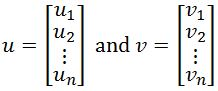Then the inner product of the two vectors will be: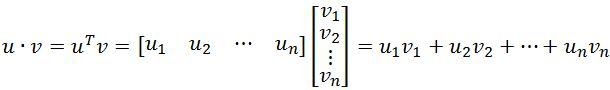Let $u,v$ and $w$ be vectors in $\Bbb{R}^n$, and let $c$ be a scalar. Then,
1) $u \cdot v=v \cdot u$
2) $w(u+v)=w \cdot u+w \cdot v$
3) $(cu) \cdot v=c(u \cdot v)=u \cdot (cv)$
4) $u \cdot u \geq 0$, and $u \cdot u=0$ only if $u=0$

Suppose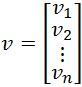. Then the length (or norm) of a vector $v$ is

$\lVert v \rVert = \sqrt{v_{1}^2+v_{2}^2+\cdots +v_{n}^2}\;,\; \lVert v \rVert ^2 = v \cdot v$

Suppose dist$(u,v)$ is the distance between the vectors $u$ and $v$. To find the distance between the two vectors, we calculate
dist$(u,v)=\lVert u-v \rVert$

If two vectors $u$ and $v$ are orthogonal to each other, then it must be true that
$u \cdot v =0$
• Introduction
Inner Product, Length, and Orthogonality Overview:
a)
The Length of a Vector
• Also known as norm
• Some properties with a length of a vector
• Unit vectors

b)
Distance of two vectors/Orthogonal Vectors
• Dist$(u,v)=\lVert u-v \rVert$
• How to tell with two vectors are orthogonal
• Orthogonal vectors with Pythagorean Theorem

• 1.
Utilizing the Inner Product
Given that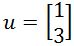and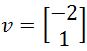, compute:
$\frac{u \cdot u}{v \cdot v}v$

• 2.
Given that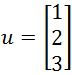and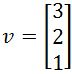, compute:
$\lVert (u \cdot v)u \rVert$

• 3.
Finding the Unit vector
Find the unit vector in the direction of the vector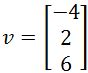• 4.
Calculating the Distance
Find the distance between the vectors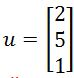and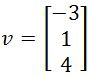.

• 5.
Showing Orthogonality
For what value(s) of $b$ make vectors $u$ and $v$ orthogonal if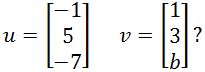• 6.
Proof Questions
Show that the parallelogram is true for vectors $u$ and $v$ in R^n. In other words, show that:
$\lVert u+v \rVert^2 + \lVert u-v \rVert^2 = 2 \lVert u \rVert^2 + 2 \lVert v \rVert^2$

• 7.
Suppose vector $u$ is orthogonal to vectors $x$ and $y$. Show that $u$ is also orthogonal to $x+y$.